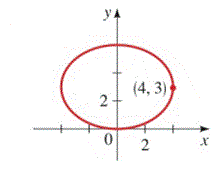Chapter 12.CT, Problem 8CT### Algebra and Trigonometry (MindTap ...

4th Edition
James Stewart + 2 others
ISBN: 9781305071742

#### Solutions

Chapter
Section### Algebra and Trigonometry (MindTap ...

4th Edition
James Stewart + 2 others
ISBN: 9781305071742
Textbook Problem

# 7-9 ■ Find an equation for the conic whose graph is shown.To determine

To find:

An equation for the conic whose graph is given.

Explanation

Given:

The graph

Approach:

Use the standard equation of the shifted ellipse (xh)2a2+(yk)2b2=1.

Calculation:

In the graph given that major axis of ellipse is parallel to the x-axis and one vertex of the ellipse is (4,3). The minor axis of the ellipse is lying on the y-axis, so the center of the ellipse will lie on the y-axis and the center will be (0,3). Distance between vertex and the center is 4 units, so a=4 and from the graph we can write the length of semi minor axis is b=3 units

### Still sussing out bartleby?

Check out a sample textbook solution.

See a sample solution

#### The Solution to Your Study Problems

Bartleby provides explanations to thousands of textbook problems written by our experts, many with advanced degrees!

Get Started